## "About One Method of Calculation of Resistance of Two-dimensional Infinite Grid Systems"

Igor Feliksovich Spivak-Lavrov*, Maksat Serikbayuly Kurmanbay, Аidana N. Mazhit

Department of Physics, Aktobe Regional State University named after K. Zhubanov, Аktobe, Kazakhstan

*Corresponding author: Igor Feliksovich Spivak-Lavrov, Department of Physics, Aktobe Regional State University named after K. Zhubanov, Аktobe, Kazakhstan. Tel: +787012503562; Email: spivakif@rambler.ru

Received Date: 07 August, 2018; Accepted Date: 24 August, 2018; Published Date: 03 September, 2018

Precise solutions of problems on finding the electrical resistance between adjacent nodes of infinite two-dimensional grids with square and triangular cells are obtained in the work. The calculation technique used is based on the use of equivalent circuits of infinite resistance chains. It is shown that the method of calculating the resistance of such systems, described in the collection of tasks, Irodov I.E. and based on the use of the principles of superposition and symmetry is only approximate. The discrepancy with the resistance values given in the collection of Irodov I.E. is about 10%.

Keywords: Infinite two-dimensional network of resistances; Resistance calculation

1. Introduction

Calculation of the resistance of complex resistor connections has always attracted the attention of physicists. Thus, they developed original methods for calculating infinite chains of resistances. At present, in connection with the development of nanotechnology, the problems of calculating the resistances of infinite resistor networks have become especially topical. Let us dwell in more detail on one problem placed in the collection . «There is a boundless wire mesh with square cells (рис. 1). The resistance of each conductor between neighboring grid nodes is r. Find the resistance of this grid between two adjacent grid nodes A and B». In the 2003 collection, this task is numbered 3.154. To this problem is given the Instruction: "To use the principles of symmetry and superposition".

In the collection  the solution of this problem is given. We will quote it below. "Connect mentally to points A and B the voltage source U. Then, where current in the supply wires, is the current in the conductor АВ. The current can be represented as a superposition of two currents. If the current "flowed into" point А and spread out along the grid to infinity, then the conductor АВ – from symmetry – would go current. Similarly, if the current entered the grid from infinity and "flowed out" of point В, then the conductor АВ would also have a current. By imposing these decisions on each other, we get therefore " (Figure 1).

2. Research Hypothesis

But this solution is only approximate, because it is obtained from the principles of superposition and symmetry, which in this case are performed only approximately. Indeed, from the same superposition principle it follows that when the current "flows into" point А, then a voltage must be applied to this point. Similarly, a voltage is applied to point В. If we apply both these voltages simultaneously, then the symmetry in the distribution of the currents at points A and B is violated because of the mutual influence of the potentials and the response of the problem will change. Moreover, it can be said that the mutual influence of potentials will lead to the fact that the current in the conductor АВ will be greater than and, consequently, the resistance.

2.1. Calculation of the Resistance of Grid with Square Cells

We give below an alternative solution to this problem. First, we divide the entire plane into two identical half-planes, cutting the grid along a straight line passing through points A and B, to the left of point A and to the right of B. For this, each of the resistances r, lying to the left of point А and to the right of В, is replaced by two parallel-connected resistance of each. We obtain the following picture, shown in Figure 2. In Figure 3 shows an equivalent scheme of a cut mesh, where the resistance of the half-planes obtained as a result of cutting is denoted by R:

Then the resistance between adjacent nodes A and B of an infinite two-dimensional grid with square cells is equal to: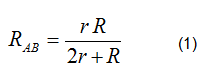To do this, we similarly cut the grid along a straight line passing through points C and D. The resulting picture is shown in Figure 4, where you can see two infinite half-planes with resistance R, located below points A, B and above points C, D, and also two identical infinite chains going to the left of points A and C and to the right of points B and D. We denote the resistance of such an infinite chain by r*. Then, comparing the schemes in Figures 4, we can draw up the equivalent circuit depicted in Figure 5.

Using the equivalent circuit in Figure 5, we write the equation for determining the resistance of the half-plane R: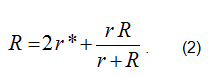Solving this quadratic equation with respect to R, we find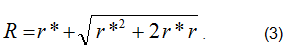The resistance of an infinite periodic chain r* is found by the standard method, solving equation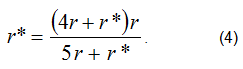Location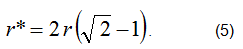Substituting (5) into (3), we obtain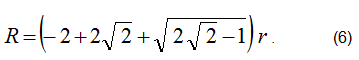Substitution of this value of R into (1) leads to the final result: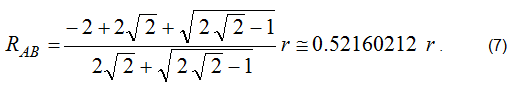This result, although not very strong, is still different from the result of 0.5 r obtained in the approximation of the principles of symmetry and superposition. In this connection it is interesting to look at the distribution of currents at point A. If current I approaches the point A, then it will be distributed as follows: according to the resistance going up and down from point A, the same currents will go, according to the resistance between nodes A and B, there will be a current, and in the opposite direction there will be a current. It's a pity, the beauty is gone, the symmetry has disappeared, and everything has become very prosaic. Well, in life it often happens that beauty deceives us and then it is difficult to get rid of beautiful illusions.

2.1. Calculation of the Resistance of Grid with Triangular Cells

Here we also give a solution to the problem of an infinite net by the same resistances r forming regular triangles, as shown in Figure 6. Suppose it is necessary to find the resistance RAB of the lattice between the two nearest nodes A and B. Using for the solution of the problem the principles of symmetry and superposition, by analogy with a square grid, it is easy to obtain the following simple result of, which is also only approximate.

In Figure 6 shows the part of the grid and the bold lines show the directions along which it is necessary to make cuts in order to break it into the same half-planes and endless chains. First, we cut along the rays issuing from the nodes A and B, thus breaking the entire grid into two half-planes with the same resistances R. As a result, just as in the case of a rectangular grid, we obtain the equivalent circuit shown in Figure 3, and formula (1) for the resistance of an infinite net.

Qn figure 6 each side of the triangle has a resistance r. The thick lines indicate the directions of the sections. To determine the resistance of the half-plane R, we cut once more the lattice along the rays emanating from the nodes C and D. We obtain the equivalent circuit shown in Figure 7 and, respectively, equation (8):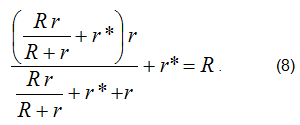After simple transformations, we obtain the following quadratic equation for the determination of R: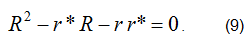Location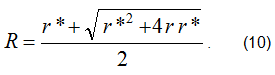Here, the resistance of the infinite chain r* is found from the equivalent circuit shown in Figure 8, which leads to the equation (11).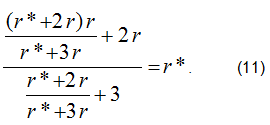Solving this equation, we find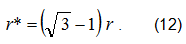Substituting this value of r* into formula (10), we obtain for R the expression: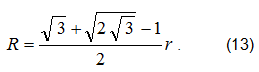Substitution of this expression in (1) leads to the final result: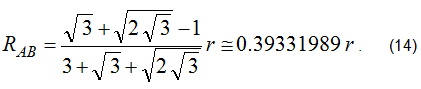3. Conclusion

Thus, in this paper we develop a method for calculating infinite networks of resistances, which makes it possible to find the exact resistance between the nearest nodes of such networks. It is shown that the calculation method used in , based on the principles of superposition and symmetry, gives only an approximate underestimated result for this resistance. And for a grid with square cells, the difference of results does not exceed 5%, and for a network with triangular elements it approaches 20%.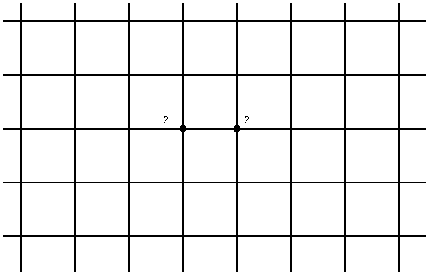Figure 1: Infinite wire mesh with square cells.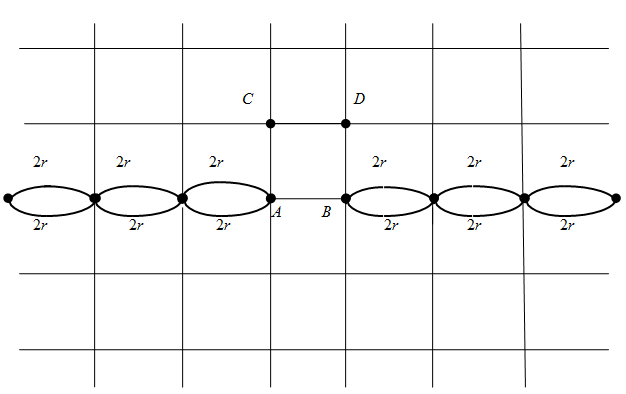Figure 2: Infinite wire mesh, divided into two half-planes.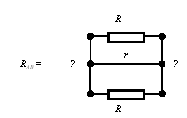Figure 3: Equivalent scheme of unlimited wire mesh.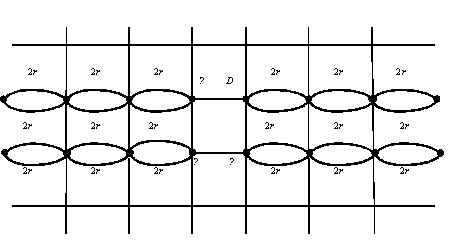Figure 4: Infinite wire mesh, cut along two straight lines.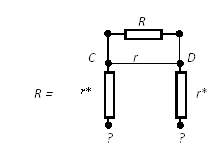Figure 5: An equivalent circuit for the resistance of the half-plane R in the case of a square grid.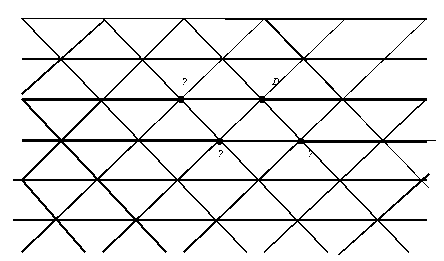Figure 6: Infinite grid of regular triangles.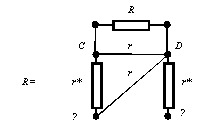Figure 7: An equivalent circuit for the resistance of the half-plane R in the case of a triangular grid.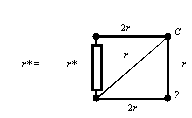Figure 8: Equivalent circuit for calculation of resistance r* infinite chain in the case of a triangular grid.

1.     Irodov IE (2003) Problems in general physics. Tutorial. 6 th ed., Sr. – SPb . Izd. "Lan", 2003.Pg. No. 416.

2.     Bessonov LA (2002) Theoretical bases of electrical engineering. Electric circuits. – Moscow: Gardariki, 2002. Pg. No. 638.

Citation: Spivak-Lavrov IF, Kurmanbai MS, Mazhit АN (2018) About One Method of Calculation of Resistance of Two-dimensional Infinite Grid Systems. Educ Res Appl: ERCA-157. DOI: 10.29011/2575-7032/100057